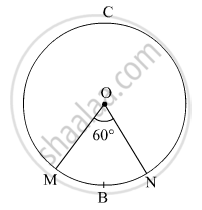Share

# In the Given Figure, the Radius of the Circle is 7 Cm and M ( Arc Mbn) = 60°, Find A(O - Mcn). - Geometry

ConceptPerimeter and Area of a Circle

#### Question

In the given figure, the radius of the circle is 7 cm and m ( arc MBN) = 60°,
find  A(O - MCN).#### Solution

Radius of the circle, r = 7 cm
m(arc MBN) = ∠MON = θ = 60º

A(O-MCN) = Area of the sector OMCN
= Area of the circle − Area of the sector OMBN
= 154 − 25.7
= 128.3 cm2

Taking Question image data : m(arcMBN) = 68°

A(O - MBN)  = (68/360)  × area of circle

= 29.09 cm²

A(O - MCN)  = area of circle  - A(O - MBN)

=> A(O - MCN)  = 154- 29.09 = 124.91 cm²

Is there an error in this question or solution?

#### APPEARS IN

Balbharati Solution for Balbharati Class 10 Mathematics 2 Geometry (2018 to Current)
Chapter 7: Mensuration
Practice set 7.3 | Q: 6.3 | Page no. 154

#### Video TutorialsVIEW ALL 

Solution In the Given Figure, the Radius of the Circle is 7 Cm and M ( Arc Mbn) = 60°, Find A(O - Mcn). Concept: Perimeter and Area of a Circle.
S